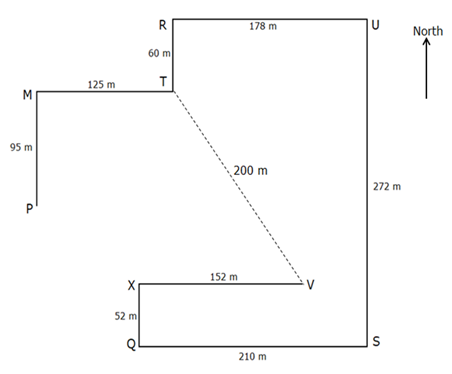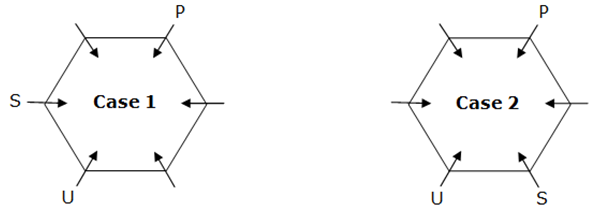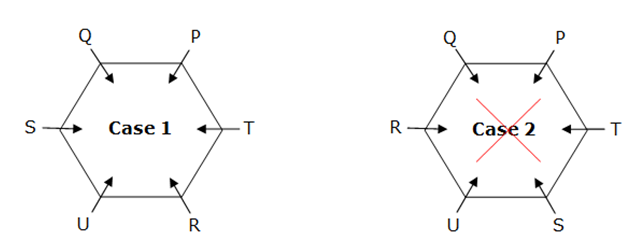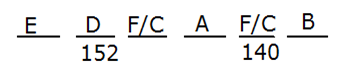# SBI Clerk Prelims Reasoning Ability Questions 2021 (Day-41)

Dear Aspirants, Our IBPS Guide team is providing new series of Reasoning Questions for SBI Clerk Prelims 2021 so the aspirants can practice it on a daily basis. These questions are framed by our skilled experts after understanding your needs thoroughly. Aspirants can practice these new series questions daily to familiarize with the exact exam pattern and make your preparation effective.

Start Quiz

Direction sense

Direction (1-3): Study the following information carefully and answer the questions given below it.

There are nine players standing on a ground. S is 210 m east of Q. R is 178 m west of U. M is 95 m north of P. U is 272 m north of S. Q is 52 m south of X. M is 125 m west of T. R is 60 m north of T. X is 152 m west of V.

1) What is the shortest distance between T and V?

A.2000 m

B.200 m

C.400 m

D.120 m

E.None of these

2) What is the direction of P with respect to X?

A.South-West

B.South-East

C.North-West

D.North-East

E.None of these

3) If a player Z is standing exactly between R and U then what will be the direction of V with respect to Z?

A.South-East

B.South-West

C.East

D.North

E.None of these

Seating

Direction (4-7): Study the following information carefully and answer the questions given below.

Six persons P, Q, R, S, T and U are seated around a hexagonal table. Each person is seated at the corner of the hexagon and all of them are facing towards the centre of the table. Two persons sit between U and P either in clockwise or anti-clockwise direction. Only one person sits between P and S. Q sits second to the right of T. R is not an immediate neighbor of Q.

4) What is the position of R with respect to U?

A.Immediate left

B.Immediate right

C.Second to the right

D.Second to the left

E.None of these

5) Who among the following persons sit exactly opposite to T?

A.U

B.R

C.P

D.S

E.Q

6) How many persons are seated between P and Q, when counted from the left of P?

A.None

B.1

C.2

D.3

E.More than three

7) Who sits exactly between R and P, when counted from left of P?

A.Q

B.S

C.T

D.U

E.None of these

Order and ranking

Direction (8-10): Study the following information carefully and answer the below questions.

There are six persons A-F having different heights are arranged descending order from left to right but not necessarily in the same order. Only two persons are shorter than A. D is taller than B but shorter than E. D is the second tallest person. Both F and C taller are than B. One who is second tallest is 152cm and one who is second shortest is 140cm.

8) If F is taller than C then who among the following is a third tallest person?

A.F

B.C

C.D

D.Either A or B

E.None of these

9) What may be the height of E?

A.151

B.156

C.150

D.149

E.None of these

10) Who is the tallest person?

A.D

B.C

C.F

D.E

E.None of these

Directions (1-3) :Directions (4-7) :Two persons sit between U and P either in clockwise or anti-clockwise direction. Only one person sits between P and S.Q sits second to the right of T. R is not an immediate neighbor of Q.Since R is placed next to Q, Case 2 gets cancelled.

Directions (8-10) :